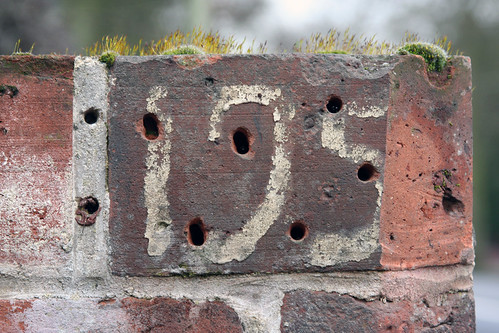# Finding Missing Values from an ArrayMissing Numbers

Today a developer came up to me and wanted help solving a problem he was running into with a list of numbers.  He had a list of 53 numbers in a comma separated list.

Unfortunately, the 53 numbers were not all in the table that he was querying.   He wanted to know if there was a quick way to find out which numbers were in the list of values and not in the table.

## The Setup

To start, the developer was running a query similar to the query below.

```USE AdventureWorks
GO

SELECT SalesOrderID
WHERE SalesOrderID IN (75120, 75121, 75122, 75123, 75124, 75125)
```

This query is bringing back all values from 75120 to 75125.  Nice and simple.  The results are as such:As the results show, though, not so nice and simple.  There are only 4 rows returned for 6 values that were included in the array of values.

In this example, it is easy to determine that the values 75124 and 75125 did not return in the results.  The developer wanted to know how he could easily find out the missing values for an array that had 53 values and 3 that didn’t return any rows.

## A Solution

To solve this problem, I showed him how to use a solution that included the use of a numbers table.  Since there wasn’t a numbers table on hand, I opted to use a technique that Itzik Ben-Gan discusses in this SQL Mag article.

With this numbers table, the array of values provided could be changed from an array of values to a result set.  The following query provides this step:

```USE AdventureWorks
GO

WITH
l0 AS (SELECT 0 AS C UNION ALL SELECT 0),
l1 AS (SELECT 0 AS C FROM L0 AS A CROSS JOIN L0 AS B),
l2 AS (SELECT 0 AS C FROM l1 AS A CROSS JOIN l1 AS B),
l3 AS (SELECT 0 AS C FROM l2 AS A CROSS JOIN l2 AS B),
l4 AS (SELECT 0 AS C FROM l3 AS A CROSS JOIN l3 AS B),
l5 AS (SELECT 0 AS C FROM l4 AS A CROSS JOIN l4 AS B),
nums AS (SELECT ROW_NUMBER() OVER(ORDER BY (SELECT NULL)) AS N FROM l5)
SELECT TOP (100000) n
FROM nums
WHERE n IN (75120, 75121, 75122, 75123, 75124, 75125)
```

The results of which are as follows:Great, the numbers from the array are in a table.  Take these and compare them back to the table with a NOT IN clause.  The query then becomes the following:

```USE AdventureWorks
GO

WITH
l0 AS (SELECT 0 AS C UNION ALL SELECT 0),
l1 AS (SELECT 0 AS C FROM L0 AS A CROSS JOIN L0 AS B),
l2 AS (SELECT 0 AS C FROM l1 AS A CROSS JOIN l1 AS B),
l3 AS (SELECT 0 AS C FROM l2 AS A CROSS JOIN l2 AS B),
l4 AS (SELECT 0 AS C FROM l3 AS A CROSS JOIN l3 AS B),
l5 AS (SELECT 0 AS C FROM l4 AS A CROSS JOIN l4 AS B),
nums AS (SELECT ROW_NUMBER() OVER(ORDER BY (SELECT NULL)) AS N FROM l5)
SELECT TOP (100000) n
FROM nums
WHERE n IN (75120, 75121, 75122, 75123, 75124, 75125)
AND n NOT IN (SELECT SalesOrderID FROM Sales.SalesOrderHeader)
```

And the results are then the following:Viola!  A list of the two values in the array that were not in the table.

A bit of overkill when looking at an array of 6 values.  But when looking at arrays with 53 or more values eyeing it up just isn’t feasible.  A quick solution like the one provided above can be just what the doctor ordered.

## 2 thoughts on “Finding Missing Values from an Array”

1. Pingback: SQL Server Central